## Identify Linear Models Using System Identification App

### Introduction

#### Objectives

Estimate and validate linear models from single-input/single-output (SISO) data to find the one that best describes the system dynamics.

After completing this tutorial, you will be able to accomplish the following tasks using the System Identification app:

• Import data arrays from the MATLAB® workspace into the app.

• Plot the data.

• Process data by removing offsets from the input and output signals.

• Estimate, validate, and compare linear models.

• Export models to the MATLAB workspace.

### Note

The tutorial uses time-domain data to demonstrate how you can estimate linear models. The same workflow applies to fitting frequency-domain data.

This tutorial is based on the example in section 17.3 of System Identification: Theory for the User, Second Edition, by Lennart Ljung, Prentice Hall PTR, 1999.

#### Data Description

This tutorial uses the data file `dryer2.mat`, which contains single-input/single-output (SISO) time-domain data from Feedback Process Trainer PT326. The input and output signals each contain 1000 data samples.

This system heats the air at the inlet using a mesh of resistor wire, similar to a hair dryer. The input is the power supplied to the resistor wires, and the output is the air temperature at the outlet.

### Preparing Data for System Identification

Load the data in `dryer2.mat` by typing the following command in the MATLAB Command Window:

```load dryer2 ```

This command loads the data into the MATLAB workspace as two column vectors, `u2` and `y2`, respectively. The variable `u2` is the input data and `y2` is the output data.

#### Opening the System Identification App

To open the System Identification app, type the following command in the MATLAB Command Window:

`systemIdentification`

The default session name, `Untitled`, appears in the title bar.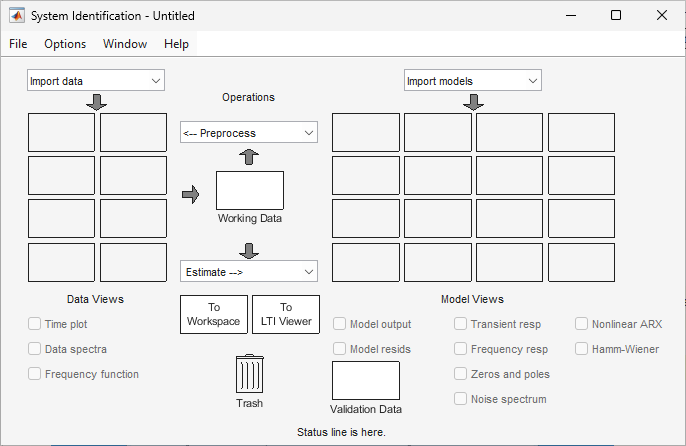#### Importing Data Arrays into the System Identification App

You can import the single-input/single-output (SISO) data from a sample data file `dryer2.mat` into the app from the MATLAB workspace.

You must have already loaded the sample data into MATLAB, as described in Loading Data into the MATLAB Workspace, and opened the System Identification app, as described in Opening the System Identification App.

If you have not performed these steps, click here to complete them.

To import data arrays into the System Identification app:

1. Select Import data > Time domain data. This action opens the Import Data dialog box.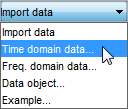2. In the Import Data dialog box, specify the following options:

• Input — Enter `u2` as the name of the input variable.

• Output — Enter `y2` as the name of the output variable.

• Data name — Change the default name to `data`. This name labels the data in the System Identification app after the import operation is completed.

• Starting time — Enter `0` as the starting time. This value designates the starting value of the time axis on time plots.

• Sample Time — Enter `0.08` as the time between successive samples in seconds. This value is the actual sample time in the experiment.

The Import Data dialog box now resembles the following figure.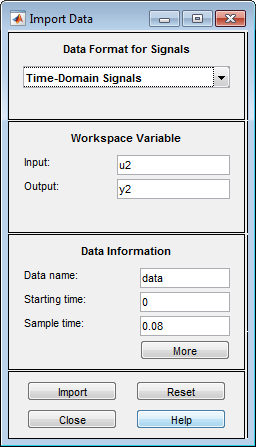3. In the Data Information area, click to expand the dialog box and specify the following options:

Input Properties

• InterSample — Accept the default `zoh` (zero-order hold) to indicate that the input signal was piecewise-constant between samples during data acquisition. This setting specifies the behavior of the input signals between samples when you transform the resulting models between discrete-time and continuous-time representations.

• Period — Accept the default `inf` to specify a nonperiodic input.

### Note

For a periodic input, enter the whole number of periods of the input signal in your experiment.

Channel Names

• Input — Enter `power`.

### Tip

Naming channels helps you to identify data in plots. For multivariable input and output signals, you can specify the names of individual Input and Output channels, separated by commas.

• Output — Enter `temperature`.

Physical Units of Variables

• Input — Enter `W` for power units.

### Tip

When you have multiple inputs and outputs, enter a comma-separated list of Input and Output units corresponding to each channel.

• Output — Enter `^oC` for temperature units.

Notes — Enter comments about the experiment or the data. For example, you might enter the experiment name, date, and a description of experimental conditions. When you estimate models from this data, these models inherit your notes.

The expanded Import Data dialog box now resembles the following figure.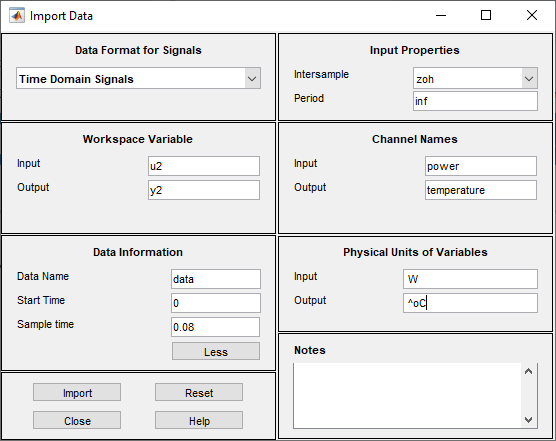4. Click to add the data to the System Identification app. The app displays an icon to represent the data.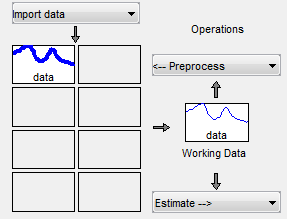5. Click to close the Import Data dialog box.

#### Plotting and Processing Data

In this portion of the tutorial, you evaluate the data and process it for system identification. You learn how to:

• Plot the data.

• Remove offsets from the data by subtracting the mean values of the input and the output.

• Split the data into two parts to use one part model estimation and the other part for model validation.

The reason you subtract the mean values from each signal is because, typically, you build linear models that describe the responses for deviations from a physical equilibrium. With steady-state data, it is reasonable to assume that the mean levels of the signals correspond to such an equilibrium. Thus, you can seek models around zero without modeling the absolute equilibrium levels in physical units.

You must have already imported data into the System Identification app, as described in Importing Data Arrays into the System Identification App.

If you have not performed this step, click here to complete it.

To plot and process the data:

1. Select the Time plot check box to open the Time Plot. If the plot window is empty, click the `data` icon in the System Identification app.The top axes show the output data (temperature), and the bottom axes show the input data (power). Both the input and the output data have nonzero mean values.

2. Subtract the mean input value from the input data and the mean output value from the output data. In the System Identification app, select <--Preprocess > Remove means.This action adds a new data set to the System Identification app with the default name `datad` (the suffix d means detrend), and updates the Time Plot window to display both the original and the detrended data. The detrended data has a zero mean value.3. Specify the detrended data to be used for estimating models. Drag the data set `datad` to the Working Data rectangle.Alternatively, right-click the `datad` icon to open the Data/model Info dialog box.Select the Use as Working Data check-box. Click and then . This action adds `datad` to the Working Data rectangle.

4. Split the data into two parts and specify the first part for model estimation, and the second part for model validation.

1. Select <--Preprocess > Select range to open the Select Range window.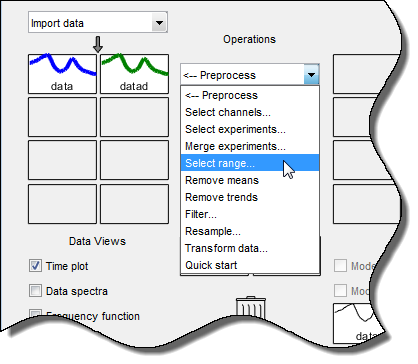2. In the Select Range window, create a data set containing the first 500 samples. In the Samples field, specify `1 500`.

### Tip

You can also select data samples using the mouse by clicking and dragging a rectangular region on the plot. If you select samples on the input-channel axes, the corresponding region is also selected on the output-channel axes.

3. In the Data name field, type the name `data_est`.4. Click to add this new data set to the System Identification app to be used for model estimation.

5. Repeat this process to create a second data set containing a subset of the data to use for validation. In the Select Range window, specify the last 500 samples in the Samples field. Type the name `data_val` in the Data name field. Click to add this new data set to the System Identification app.6. Click to close the Select Range window.

5. In the System Identification app, drag and drop `data_est` to the Working Data rectangle, and drag and drop `data_val` to the Validation Data rectangle.6. To get information about a data set, right-click its icon. For example, right-click `data_est` to open the Data/model Info dialog box.You can also change certain values in the Data/model Info dialog box, including:

• Changing the name of the data set in the Data name field.

• Changing the color of the data icon in the Color field. You specify colors using RGB values (relative amounts of red, green, and blue). Each value is between `0` and `1`. For example, `[1,0,0]` indicates that only red is present, and no green and blue are mixed into the overall color.

• Viewing or editing the commands executed on this data set in the Diary and Notes area. This area contains the command-line equivalent to the processing you performed using the System Identification app. For example, as shown in the Data/model Info: estimate window, the `data_est` data set is a result of importing the data, detrending the mean values, and selecting the first 500 samples of the data.

``` % Import data datad = detrend(data,0) data_est = datad([1:500])```

The Data/model Info dialog box also displays the total number of samples, the sample time, and the output and input channel names and units. This information is not editable.

### Tip

As an alternative shortcut, you can select Preprocess > Quick start from the System Identification app to perform all of the data processing steps in this tutorial.

Learn More.  For information about supported data processing operations, such as resampling and filtering the data, see Preprocess Data.

### Saving the Session

After you process the data, as described in Plotting and Processing Data, you can delete any data sets in the window that you do not need for estimation and validation, and save your session. You can open this session later and use it as a starting point for model estimation and validation without repeating these preparatory steps.

You must have already processed the data into the System Identification app, as described in Plotting and Processing Data.

To delete specific data sets from a session and save the session:

1. In the System Identification app:

1. Drag and drop the `data` data set into Trash.

2. Drag and drop the `datad` data set into Trash.

Alternatively, you can press the key on your keyboard to move the data sets to Trash.

### Note

Moving items to the Trash does not delete them. To permanently delete items, select Options > Empty trash.

The following figure shows the System Identification app after moving the items to Trash.2. Drag and drop the `data_est` and `data_val` data sets to fill the empty rectangles, as shown in the following figure.3. Select File > Save session as to open the Save Session dialog box, and browse to the folder where you want to save the session file.

4. In the File name field, type the name of the session `dryer2_processed_data`, and click . The resulting file has a `.sid` extension.

### Tip

You can open a saved session when starting the System Identification app by typing the following command at the MATLAB prompt:

`systemIdentification('dryer2_processed_data')`

### Estimating Linear Models Using Quick Start

#### How to Estimate Linear Models Using Quick Start

You can use the Quick Start feature in the System Identification app to estimate linear models. Quick Start might produce the final linear models you decide to use, or provide you with information required to configure the estimation of accurate parametric models, such as time constants, input delays, and resonant frequencies.

You must have already processed the data for estimation, as described in Plotting and Processing Data.

If you have not performed this step, click here to complete it.

In the System Identification app , select Estimate > Quick start.

This action generates plots of step response, frequency-response, and the output of state-space and polynomial models. For more information about these plots, see Validating the Quick Start Models.#### Types of Quick Start Linear Models

Quick Start estimates the following four types of models and adds the following to the System Identification app with default names:

• `imp` — Step response over a period of time using the `impulseest` algorithm.

• `spad` — Frequency response over a range of frequencies using the `spa` algorithm. The frequency response is the Fourier transform of the impulse response of a linear system.

By default, the model is evaluated at 128 frequency values, ranging from 0 to the Nyquist frequency.

• `arxqs` — Fourth-order autoregressive (ARX) model using the `arx` algorithm.

This model is parametric and has the following structure:

y(t) represents the output at time t, u(t) represents the input at time t, na is the number of poles, nb is the number of b parameters (equal to the number of zeros plus 1), nk is the number of samples before the input affects output of the system (called the delay or dead time of the model), and e(t) is the white-noise disturbance. System Identification Toolbox™ software estimates the parameters ${a}_{1}\dots {a}_{n}$ and ${b}_{1}\dots {b}_{n}$ using the input and output data from the estimation data set.

In `arxqs`, na=nb=4, and nk is estimated from the step response model `imp`.

• `n4s3` — State-space model calculated using `n4sid`. The algorithm automatically selects the model order (in this case, 3).

This model is parametric and has the following structure:

`$\begin{array}{l}\frac{dx}{dt}=Ax\left(t\right)+Bu\left(t\right)+Ke\left(t\right)\\ y\left(t\right)=Cx\left(t\right)+Du\left(t\right)+e\left(t\right)\end{array}$`

y(t) represents the output at time t, u(t) represents the input at time t, x is the state vector, and e(t) is the white-noise disturbance. The System Identification Toolbox product estimates the state-space matrices A, B, C, D, and K.

### Note

The Quick Start option does not create a transfer function model or a process model which can also be good starting model types.

#### Validating the Quick Start Models

Quick Start generates the following plots during model estimation to help you validate the quality of the models:

• Step-response plot

• Frequency-response plot

• Model-output plot

You must have already estimated models using Quick Start to generate these plots, as described in How to Estimate Linear Models Using Quick Start.

If you have not performed this step, click here to complete it.

Step-Response Plot.  The following step-response plot shows agreement among the different model structures and the measured data, which means that all of these structures have similar dynamics.

### Tip

If you closed the plot window, select the Transient resp check box to reopen this window. If the plot is empty, click the model icons in the System Identification app window to display the models on the plot.

Step Response for imp, arxqs, and n4s3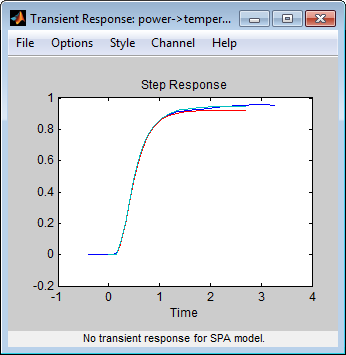### Tip

You can use the step-response plot to estimate the dead time of linear systems. For example, the previous step-response plot shows a time delay of about 0.25 s before the system responds to the input. This response delay, or dead time, is approximately equal to about three samples because the sample time is `0.08` s for this data set.

Frequency-Response Plot.  The following frequency-response plot shows agreement among the different model structures and the measured data, which means that all of these structures have similar dynamics.

### Tip

If you closed this plot window, select the Frequency resp check box to reopen this window. If the plot is empty, click the model icons in the System Identification app window to display the models on the plot.

Frequency Response for Models imp, spad, arxqs, and n4s3Model-Output Plot.  The Model Output window shows agreement among the different model structures and the measured output in the validation data.

### Tip

If you closed the Model Output window, select the Model output check box to reopen this window. If the plot is empty, click the model icons in the System Identification app window to display the models on the plot.

Measured Output and Model Output for Models imp, arxqs, and n4s3The model-output plot shows the model response to the input in the validation data. The fit values for each model are summarized in the Best Fits area of the Model Output window. The models in the Best Fits list are ordered from best at the top to worst at the bottom. The fit between the two curves is computed such that 100 means a perfect fit, and 0 indicates a poor fit (that is, the model output has the same fit to the measured output as the mean of the measured output).

In this example, the output of the models matches the validation data output, which indicates that the models seem to capture the main system dynamics and that linear modeling is sufficient.

### Tip

To compare predicted model output instead of simulated output, select this option from the Options menu in the Model Output window.

### Estimating Linear Models

#### Strategy for Estimating Accurate Models

The linear models you estimated in Estimating Linear Models Using Quick Start showed that a linear model sufficiently represents the dynamics of the system.

In this portion of the tutorial, you get accurate parametric models by performing the following tasks:

1. Identifying initial model orders and delays from your data using a simple, polynomial model structure (ARX).

2. Exploring more complex model structures with orders and delays close to the initial values you found.

The resulting models are discrete-time models.

#### Estimating Possible Model Orders

To identify black-box models, you must specify the model order. However, how can you tell what model orders to specify for your black-box models? To answer this question, you can estimate simple polynomial (ARX) models for a range of orders and delays and compare the performance of these models. You choose the orders and delays that correspond to the best model fit as an initial guess for more accurate modeling using various model structures such as transfer function and state-space models.

About ARX Models.  For a single-input/single-output system (SISO), the ARX model structure is:

y(t) represents the output at time t, u(t) represents the input at time t, na is the number of poles, nb is the number of zeros plus 1, nk is the input delay—the number of samples before the input affects the system output (called delay or dead time of the model), and e(t) is the white-noise disturbance.

You specify the model orders na, nb, and nk to estimate ARX models. The System Identification Toolbox product estimates the parameters ${a}_{1}\dots {a}_{n}$ and ${b}_{1}\dots {b}_{n}$ from the data.

How to Estimate Model Orders

1. In the System Identification app, select Estimate > Polynomial Models to open the Polynomial Models dialog box.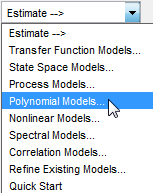2. From the Structure list, select ```ARX: [na nb nk]```. By default, this is already selected.

3. Edit the Orders field to try all combinations of poles, zeros, and delays, where each value is from 1 to 10:

`[1:10 1:10 1:10]`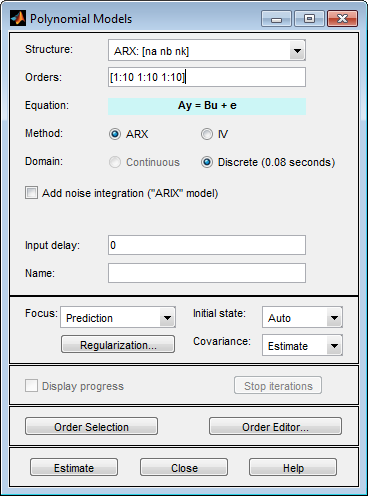4. Click to open the ARX Model Structure Selection window, which displays the model performance for each combination of model parameters.

You use this plot to select the best-fit model.• The horizontal axis is the total number of parameters — na + nb.

• The vertical axis, called Unexplained output variance (in %), is the portion of the output not explained by the model—the ARX model prediction error for the number of parameters shown on the horizontal axis.

The prediction error is the sum of the squares of the differences between the validation data output and the model one-step-ahead predicted output.

• nk is the delay.

Three rectangles are highlighted on the plot in green, blue, and red. Each color indicates a type of best-fit criterion, as follows:

• Red — Best fit minimizes the sum of the squares of the difference between the validation data output and the model output. This rectangle indicates the overall best fit.

• Green — Best fit minimizes Rissanen MDL criterion.

• Blue — Best fit minimizes Akaike AIC criterion.

In this tutorial, the Unexplained output variance (in %) value remains approximately constant for the combined number of parameters from 4 to 20. Such constancy indicates that model performance does not improve at higher orders. Thus, low-order models might fit the data equally well.

### Note

When you use the same data set for estimation and validation, use the MDL and AIC criteria to select model orders. These criteria compensate for overfitting that results from using too many parameters. For more information about these criteria, see the `selstruc` reference page.

5. In the ARX Model Structure Selection window, click the red bar (corresponding to `15` on the horizontal axis), and click . This selection inserts na=6, nb=9, and nk=2 into the Polynomial Models dialog box and performs the estimation.

This action adds the model `arx692` to the System Identification app and updates the plots to include the response of the model.

### Note

The default name of the parametric model contains the model type and the number of poles, zeros, and delays. For example, `arx692` is an ARX model with na=6, nb=9, and a delay of two samples.

6. In the ARX Model Structure Selection window, click the third bar corresponding to `4` parameters on the horizontal axis (the lowest order that still gives a good fit), and click .

• This selection inserts na=2, nb=2, and nk=3 (a delay of three samples) into the Polynomial Models dialog box and performs the estimation.

• The model `arx223` is added to the System Identification app and the plots are updated to include its response and output.

7. Click to close the ARX Model Structure Selection window.

8. Click to close the Polynomial Models dialog box.

#### Identifying Transfer Function Models

By estimating ARX models for different order combinations, as described in Estimating Possible Model Orders, you identified the number of poles, zeros, and delays that provide a good starting point for systematically exploring different models.

The overall best fit for this system corresponds to a model with six poles, nine zeros, and a delay of two samples. It also showed that a low-order model with na = 2 (two poles), nb = 2 (one zero), and nk = 3 (input-output delay) also provides a good fit. Thus, you should explore model orders close to these values.

In this portion of the tutorial, you estimate a transfer function model.

About Transfer Function Models.  The general transfer function model structure is:

`$Y\left(s\right)=\frac{num\left(s\right)}{den\left(s\right)}U\left(s\right)+E\left(s\right)$`

Y(s), U(s) and E(s) represent the Laplace transforms of the output, input and error, respectively. num(s) and den(s) represent the numerator and denominator polynomials that define the relationship between the input and the output. The roots of the denominator polynomial are referred to as the model poles. The roots of the numerator polynomial are referred to as the model zeros.

You must specify the number of poles and zeros to estimate a transfer function model. The System Identification Toolbox product estimates the numerator and denominator polynomials, and input/output delays from the data.

The transfer function model structure is a good choice for quick estimation because it requires that you specify only 2 parameters to get started: `np` is the number of poles and `nz` is the number of zeros.

How to Estimate Transfer Function Models

1. In the System Identification app, select Estimate > Transfer Function Models to open the Transfer Functions dialog box.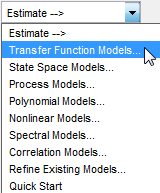2. In the Transfer Functions dialog box, specify the following options:

• Number of poles — Leave the default value `2` to specify a second order function, for two poles.

• Number of zeros — Leave the default value `1`.

• Continuous-time — Leave this checked.

3. Click I/O Delay to expand the input/output delay specification area.

By estimating ARX models for different order combinations, as described in Estimating Possible Model Orders, you identified a 3 sample delay (`nk = 3`). This delay translates to a continuous-time delay of `(nk-1)*Ts `, which is equal to 0.16 seconds.

Specify Delay as `0.16` seconds. Leave Fixed checked.

Use the default Estimation Options. By default, the app assigns the name `tf1` to the model. The dialog box should look like this.4. Click to add a transfer function model called `tf1` to the System Identification app. You can view the output of the estimation of the transfer function model in comparison with the estimations of other models, in the Model output window.### Tip

If you closed the Model Output window, you can regenerate it by selecting the Model output check box in the System Identification app. If the new model does not appear on the plot, click the model icon in the System Identification app to make the model active.

5. Click to close the Transfer Functions dialog box.

#### Identifying State-Space Models

By estimating ARX models for different order combinations, as described in Estimating Possible Model Orders, you identified the number of poles, zeros, and delays that provide a good starting point for systematically exploring different models.

The overall best fit for this system corresponds to a model with six poles, nine zeros, and a delay of two samples. It also showed that a low-order model with na=2 (two poles), nb=2 (one zero), and nk=3 (input-output delay) also provides a good fit. Thus, you should explore model orders close to these values.

In this portion of the tutorial, you estimate a state-space model.

About State-Space Models.  The general state-space model structure (innovation form) is:

`$\begin{array}{l}\frac{dx}{dt}=Ax\left(t\right)+Bu\left(t\right)+Ke\left(t\right)\\ y\left(t\right)=Cx\left(t\right)+Du\left(t\right)+e\left(t\right)\end{array}$`

y(t) represents the output at time t, u(t) represents the input at time t, x(t) is the state vector at time t, and e(t) is the white-noise disturbance.

You must specify a single integer as the model order (dimension of the state vector) to estimate a state-space model. The System Identification Toolbox product estimates the state-space matrices A, B, C, D, and K from the data.

The state-space model structure is a good choice for quick estimation because it requires that you specify only the number of states (which equals the number of poles). You can optionally also specify the delays and feedthrough behavior.

How to Estimate State-Space Models

1. In the System Identification app, select Estimate > State Space Models to open the State Space Models dialog box.2. In the Specify value field, specify the model order. Type `6` to create a sixth-order state-space model.

This choice is based on the fact that the best-fit ARX model has six poles.

### Tip

Although this tutorial estimates a sixth-order state-space model, you might want to explore whether a lower-order model adequately represents the system dynamics.

3. Click Estimation Options to expand the estimation options area.

4. Change Focus to `Simulation` to optimize the model to use for output simulation.

The State Space Models dialog box looks like the following figure.5. Click to add a state-space model called `ss1` to the System Identification app.

You can view the output of the estimation of the state-space model in comparison with the estimations of other models, in the Model output window.### Tip

If you closed the Model Output window, you can regenerate it by selecting the Model output check box in the System Identification app. If the new model does not appear on the plot, click the model icon in the System Identification app to make the model active.

6. Click to close the State Space Models dialog box.

#### Identifying ARMAX Models

By estimating ARX models for different order combinations, as described in Estimating Possible Model Orders, you identified the number of poles, zeros, and delays that provide a good starting point for systematically exploring different models.

The overall best fit for this system corresponds to a model with six poles, nine zeros, and a delay of two samples. It also showed that a low-order model with na=2 (two poles), nb=2 (one zero), and nk=3 also provides a good fit. Thus, you should explore model orders close to these values.

In this portion of the tutorial, you estimate an ARMAX input-output polynomial model.

About ARMAX Models.  For a single-input/single-output system (SISO), the ARMAX polynomial model structure is:

y(t) represents the output at time t, u(t) represents the input at time t, na is the number of poles for the dynamic model, nb is the number of zeros plus 1, nc is the number of poles for the disturbance model, nk is the number of samples before the input affects output of the system (called the delay or dead time of the model), and e(t) is the white-noise disturbance.

### Note

The ARMAX model is more flexible than the ARX model because the ARMAX structure contains an extra polynomial to model the additive disturbance.

You must specify the model orders to estimate ARMAX models. The System Identification Toolbox product estimates the model parameters ${a}_{1}\dots {a}_{n}$, ${b}_{1}\dots {b}_{n}$, and ${c}_{1}\dots {c}_{n}$ from the data.

How to Estimate ARMAX Models

1. In the System Identification app , select Estimate > Polynomial Models to open the Polynomial Models dialog box.2. From the Structure list, select ```ARMAX: [na nb nc nk]``` to estimate an ARMAX model.3. In the Orders field, set the orders na, nb, nc, and nk to the following values:

`[2 2 2 2]`

The app assigns the name to the model `amx2222`, by default, visible in the Name field.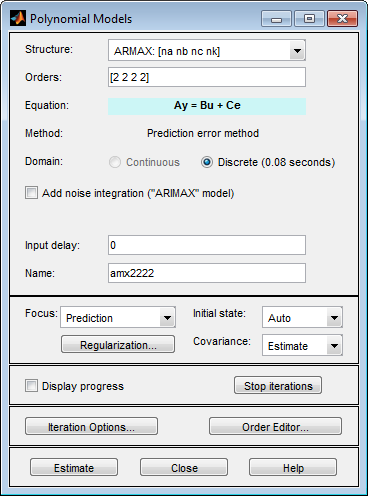4. Click to add the ARMAX model to the System Identification app.

5. Repeat steps 3 and 4 using higher Orders `3 3 2 2`. These orders result in a model that fits the data almost as well as the higher order ARX model `arx692`.### Tip

If you closed the Model Output window, you can regenerate it by selecting the Model output check box in the System Identification app. If the new model does not appear on the plot, click the model icon in the System Identification app to make the model active.

6. Click to close the Polynomial Models dialog box.

#### Choosing the Best Model

You can compare models to choose the model with the best performance.

You must have already estimated the models, as described in Estimating Linear Models.

If you have not performed this step, click here to complete it.

Summary of Models.  The following figure shows the System Identification app, which includes all the estimated models in Estimating Linear Models.Examining the Model Output.  Examine the model output plot to see how well the model output matches the measured output in the validation data set. A good model is the simplest model that best describes the dynamics and successfully simulates or predicts the output for different inputs. Models are listed by name in the Best Fits area of the Model Output plot. Note that one of the simpler models, `amx3322`, produced a similar fit as the highest-order model you created, `arx692`.### Tip

If you closed the Model Output window, you can regenerate it by selecting the Model output check box in the System Identification app. If the new model does not appear on the plot, click the model icon in the System Identification app to make the model active.

To validate your models using a different data set, you can drag and drop this data set into the Validation Data rectangle in the System Identification app. If you transform validation data into the frequency domain, the Model Output plot updates to show the model comparison in the frequency domain.

To get a closer look at how well these models fit the data, magnify a portion of the plot by clicking and dragging a rectangle around the region of interest, as shown in the following figure.Releasing the mouse magnifies this region and shows that the output of all models matches the validation data well.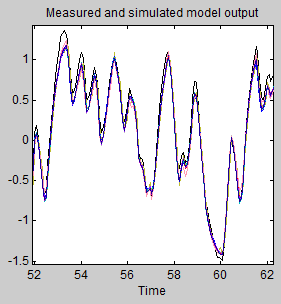### Viewing Model Parameters

#### Viewing Model Parameter Values

You can view the numerical parameter values for each estimated model.

You must have already estimated the models, as described in Estimating Linear Models.

If you have not performed this step, click here to complete it.

To view the parameter values of the model `amx3322`, right-click the model icon in the System Identification app. The Data/model Info dialog box opens.The noneditable area of the Data/model Info dialog box lists the parameter values correspond to the following difference equation for your system:

### Note

The coefficient of u(t-2) is not significantly different from zero. This lack of difference explains why delay values of both `2` and `3` give good results.

Parameter values appear in the following format:

`$\begin{array}{l}A\left(z\right)=1+{a}_{1}{z}^{-1}+\dots +{a}_{na}{z}^{-na}\\ B\left(z\right)={b}_{1}{z}^{-nk}+\dots +{b}_{nb}{z}^{-nb-nk+1}\\ C\left(z\right)=1+{c}_{1}{z}^{-1}+\dots +{c}_{nc}{z}^{-nc}\end{array}$`

The parameters appear in the ARMAX model structure, as follows:

`$A\left(q\right)y\left(t\right)=B\left(q\right)u\left(t\right)+C\left(q\right)e\left(t\right)$`

which corresponds to this general difference equation:

y(t) represents the output at time t, u(t) represents the input at time t, na is the number of poles for the dynamic model, nb is the number of zeros plus 1, nc is the number of poles for the disturbance model, nk is the number of samples before the input affects output of the system (called the delay or dead time of the model), and e(t) is the white-noise disturbance.

#### Viewing Parameter Uncertainties

You can view parameter uncertainties of estimated models.

You must have already estimated the models, as described in Estimating Linear Models.

If you have not performed this step, click here to complete it. To view the parameter values of the model `amx3322`, right-click the model icon in the System Identification app . The Data/model Info dialog box opens.

To view parameter uncertainties, click in the Data/model Info dialog box, and view the model information at the MATLAB prompt.

```amx3322 = Discrete-time ARMAX model: A(z)y(t) = B(z)u(t) + C(z)e(t) A(z) = 1 - 1.502 (+/- 0.05982) z^-1 + 0.7193 (+/- 0.0883) z^-2 - 0.1179 (+/- 0.03462) z^-3 B(z) = 0.003956 (+/- 0.001551) z^-2 + 0.06245 (+/- 0.002372) z^-3 + 0.02673 (+/- 0.005651) z^-4 C(z) = 1 - 0.5626 (+/- 0.07322) z^-1 + 0.2355 (+/- 0.05294) z^-2 Name: amx3322 Sample time: 0.08 seconds Parameterization: Polynomial orders: na=3 nb=3 nc=2 nk=2 Number of free coefficients: 8 Use "polydata", "getpvec", "getcov" for parameters and their uncertainties. Status: Termination condition: Near (local) minimum, (norm(g) < tol). Number of iterations: 5, Number of function evaluations: 11 Estimated using POLYEST on time domain data "data_est". Fit to estimation data: 95.3% (prediction focus) FPE: 0.001596, MSE: 0.001546 More information in model's "Report" property. ```

The 1-standard deviation uncertainty for the model parameters is in parentheses next to each parameter value.

### Exporting the Model to the MATLAB Workspace

The models you create in the System Identification app are not automatically available in the MATLAB workspace. To make a model available to other toolboxes, Simulink®, and System Identification Toolbox commands, you must export your model from the System Identification app to the MATLAB workspace. For example, if the model is a plant that requires a controller, you can import the model from the MATLAB workspace into the Control System Toolbox™ product.

You must have already estimated the models, as described in Estimating Linear Models.

If you have not performed this step, click here to complete it.

To export the `amx3322` model, drag it to the To Workspace rectangle in the System Identification app. Alternatively, click in the Data/model Info dialog box.The model appears in the MATLAB Workspace browser.### Note

This model is an `idpoly` model object.

After the model is in the MATLAB workspace, you can perform other operations on the model. For example, if you have the Control System Toolbox product installed, you might transform the model to a state-space object using:

`ss_model=ss(amx3322)`

### Exporting the Model to the Linear System Analyzer

If you have the Control System Toolbox product installed, the To Linear System Analyzer rectangle appears in the System Identification app.

The Linear System Analyzer is a graphical user interface for viewing and manipulating the response plots of linear models. It displays the following plots:

• Step- and impulse-response

• Bode, Nyquist, and Nichols

• Frequency-response singular values

• Pole/zero

• Response to general input signals

• Unforced response starting from given initial states (only for state-space models)

To plot a model in the Linear System Analyzer, drag and drop the model icon to the To Linear System Analyzer rectangle in the System Identification app. Alternatively, click in the Data/model Info dialog box.

For more information about working with plots in the Linear System Analyzer, see Linear System Analyzer Overview (Control System Toolbox).

##### SupportGet trial now